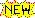The Frame Problem

 Last Update: 24 November 2008, 3:31 p.m. Note:ormaterial is highlighted

Horty, John F. (2001), "Nonmonotonic Logic", in Lou Goble (ed.), The Blackwell Guide to Philosophical Logic (Malden, MA: Blackwell): 336-361,

using some of the notation of:

1. Let s1 be a blocks-world situation, with blocks a,b,c,d and table "table1" described by:

1. On(b,a,s1)
2. Clear(b,s1)
3. Clear(c,s1)
4. Clear(d,s1)
5. On(a,table1,s1)
6. On(c,table1,s1)
7. On(d,table1,s1)

Suppose that there is a robot that can do the following actions on blocks:

1. Stack(x,y)
2. Unstack(x,y)2. Suppose that the following precondition/effect axioms apply:

1. (∀s,x,y)[Clear(x,s) ∧ Clear(y,s) ∧ x ≠ y ⊃ On(x,y,Do(Stack(x,y),s))]
2. (∀s,x,y)[On(x,y,s) ∧ clear(x,s) ⊃ Clear(y,Do(Unstack(x,y),s))]

3. Let A =def the sequence of actions A1;...;A_n.

Let Do(A,s) =def Do(A_n, Do(A1;...;A_n-1,s)).

Let KB contain descriptions of initial situation s1, the effect axioms, the definition of Do, etc.

Let φ(x,s) be a proposition expressing the goal situation.

Then "the planning problem" is to find A such that KB ||- &phi(x, Do(A,s))

4. E.g., suppose KB = I & II & III above,

& suppose φ = On(a,c,s1).

Then a plan is: A = Unstack(b,a); Stack(a,c).

I.e., show KB ||- On(a,c, Do(A,s1)).

5. But this can't be done!:

From II(2), can infer: Clear(a, Do(Unstack(b,a),s1)).

From I(3), the above "Clear" proposition, & II(1), we would like to infer:

On(a,c, Do(Stack(a,c), Do(Unstack(b,a),s1)))

But to infer that, we need:

Clear(c, Do(Unstack(b,a),s1))

But, from the fact that c is initially clear, we cannot infer that it remains clear!

That is the frame problem!